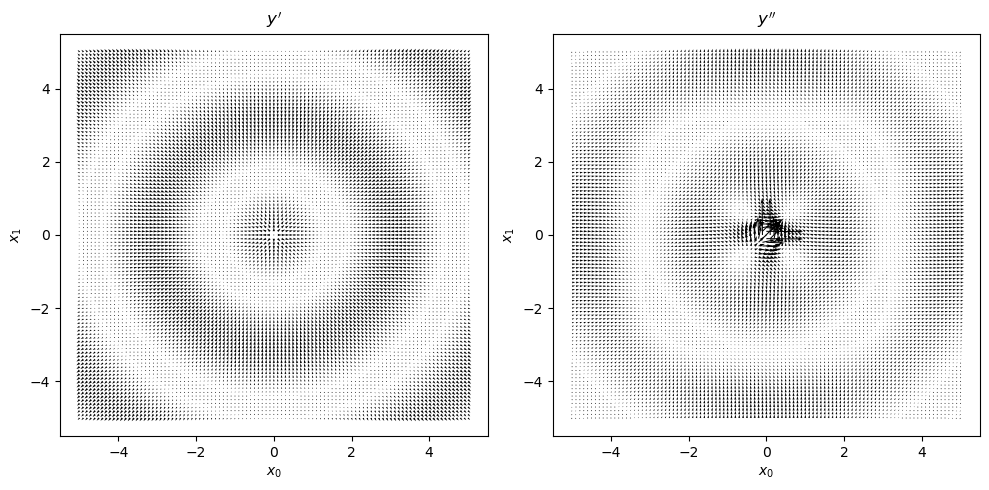For a given function, $$y = f(x)$$, differentiation of the function provides the velocity of change at a particular point (assuming $$f(x)$$ is differentiable at the point $$x$$). Differentiating a function once is called the first-order derivative and denoted $$y' = f'(x)$$. You will also see other notations denoting the first-order derivative.

• $$y' = f'(x)$$

• $$\dfrac{\mathrm{d} y}{\mathrm{d} x} = \dfrac{\mathrm{d} f(x)}{\mathrm{d} x}$$

• $$y' = \nabla f(x)$$

The value of the derivative at a particular point describes the slope of the line tangent to that point.

The second-order derivative is denoted as follows and provides the acceleration of change at a particular point.

• $$y'' = f''(x)$$

• $$\dfrac{\mathrm{d}^2 y}{\mathrm{d} x^2} = \dfrac{\mathrm{d}^2 f(x)}{\mathrm{d} x^2}$$

• $$y' = \nabla^2 f(x)$$

Being able to compute the derivative of a function and using it to evaluate the rate of change at a particular point is useful for optimization problems (eg finding the global minimum typically equates to finding a point whose tangent line has a slope of zero).

When there are more than one input variable, the gradient generalizes differentiation for the multivariable case. For example, assume we have the following function.

• $$y = x_0^2 + x_1^2$$

We would like to know how $$y$$ changes with respect to $$x_0$$, $$\dfrac{\partial y}{\partial x_0}$$, and also with respect to $$x_1$$, $$\dfrac{\partial y}{\partial x_1}$$.

• $$\dfrac{\partial y}{\partial x_0} = 2 x_0$$

• $$\dfrac{\partial y}{\partial x_1} = 2 x_1$$

The second order partial derivatives would look like the following.

• $$\dfrac{\partial^2 y}{\partial x_0^2} = 2$$

• $$\dfrac{\partial^2 y}{\partial x_1^2} = 2$$

Let’s have fun and try to compute the first and second order derivatives for some functions. We will use numdifftools to evaluate the derivatives and gradients of the following functions.

• $$y = f(x) = x^2$$

• $$y = f(x) = \sin(x)$$

• $$y = f(x) = e^{-x}$$

• $$y = f(x) = \tanh(x)$$

• $$y = f(x_0, x_1) = \sin \left( \sqrt{x_0^2 + x_1^2} \right)$$

## 7.1. $$y = f(x) = x^2$$

This function is a parabola. As you can see, $$y'$$ slopes up while $$y''$$ is constant.

:

import pandas as pd
import numpy as np
import numdifftools as nd
import matplotlib.pyplot as plt

def get_derivatives(f, x_min=-10, x_max=10, x_step=0.01):
return pd.DataFrame({'x': np.arange(x_min, x_max + x_step, x_step)}) \
.assign(**{
"y": lambda d: f(d['x']),
"y'": lambda d: nd.Derivative(f, n=1)(d['x']),
"y''": lambda d: nd.Derivative(f, n=2)(d['x'])
})

def plot_derivatives(df):
fig, ax = plt.subplots(1, 2, figsize=(10, 3))

df \
.set_index(['x'])['y'] \
.plot(kind='line', ax=ax, ylabel='y', title=r'$y = f(x)$')

df \
.set_index(['x'])[["y'", "y''"]] \
.plot(kind='line', ax=ax, title=r"$y'$ and $y''$")

fig.tight_layout()

:

_temp = get_derivatives(lambda x: x ** 2)
plot_derivatives(_temp)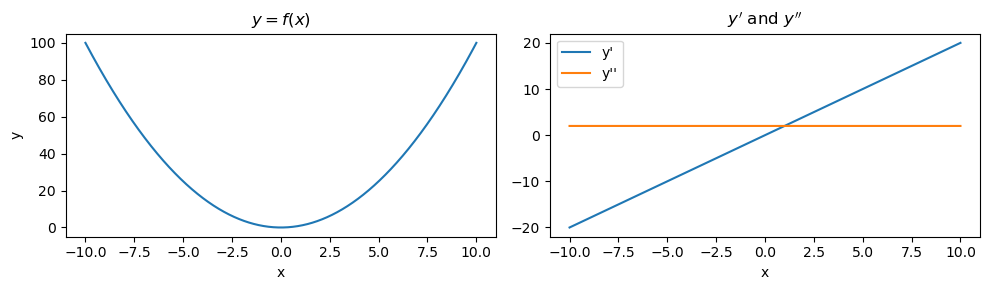## 7.2. $$y = f(x) = \sin(x)$$

This function is a sine wave. As you can see $$y'$$ and $$y''$$ are also sinusoidal and nearly out of phase.

:

_temp = get_derivatives(lambda x: np.sin(x))
plot_derivatives(_temp)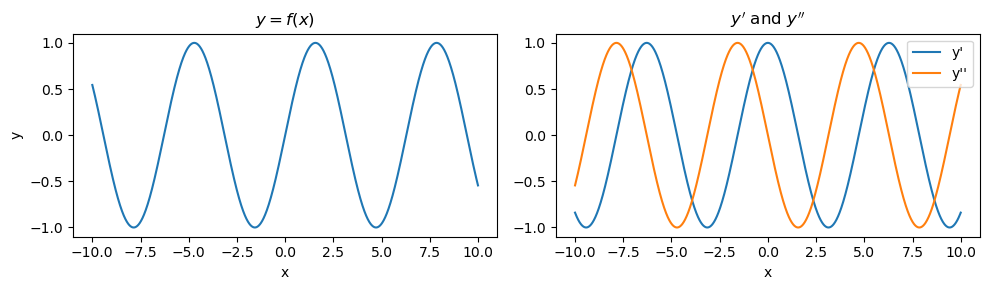## 7.3. $$y = f(x) = e^{-x}$$

This function is an exponential function. Note that $$y'$$ increases exponentially and $$y''$$ decreases exponentially.

:

_temp = get_derivatives(lambda x: np.exp(-x))
plot_derivatives(_temp)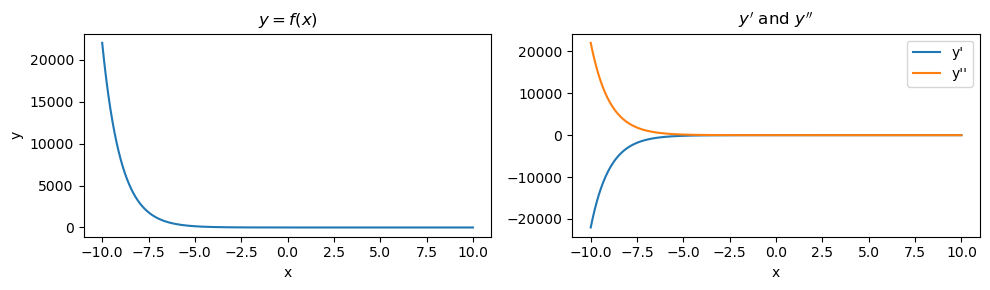## 7.4. $$y = f(x) = \tanh(x)$$

This function is the hyperbolic tangent function. For a certain range, $$y'$$ and $$y''$$ go in opposite ways.

:

_temp = get_derivatives(lambda x: np.tanh(x))
plot_derivatives(_temp)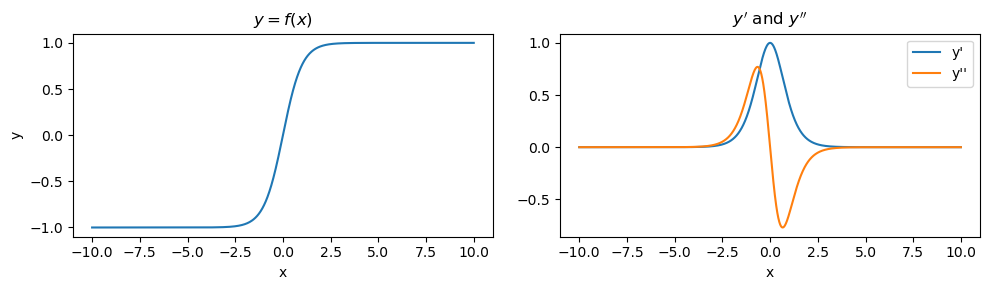## 7.5. $$y = f(x_0, x_1) = \sin \left( \sqrt{x_0^2 + x_1^2} \right)$$

At last, we are at a multivariable function. To visualize the gradients, we will use gradient field visualizations as below.

:

f = lambda x: np.sin(np.sqrt(np.power(x, 2.0) + np.power(x, 2.0)))

x0, x1 = np.meshgrid(np.arange(-5, 5.1, 0.1), np.arange(-5, 5.1, 0.1))
print(f'{x0.shape=}, {x1.shape=}')

x0, x1 = np.ravel(x0), np.ravel(x1)
print(f'{x0.shape=}, {x1.shape=}')

_df = pd.DataFrame({
'x0': x0,
'x1': x1
}) \
.assign(**{
'y': lambda d: d.apply(f, axis=1),
"y'": lambda d: d[['x0', 'x1']].apply(lambda r: f1(r), axis=1),
"y''": lambda d: d[['x0', 'x1']].apply(lambda r: f2(r), axis=1),
'g10': lambda d: d[["y'"]].apply(lambda r: r, axis=1),
'g11': lambda d: d[["y'"]].apply(lambda r: r, axis=1),
'g20': lambda d: d[["y''"]].apply(lambda r: r, axis=1),
'g21': lambda d: d[["y''"]].apply(lambda r: r, axis=1)
}) \
.drop(columns=["y'", "y''"])

x0.shape=(101, 101), x1.shape=(101, 101)
x0.shape=(10201,), x1.shape=(10201,)

:

fig, ax = plt.subplots(1, 2, figsize=(10, 5))

ax.quiver(_df['x0'], _df['x1'], _df['g10'], _df['g11'])
ax.quiver(_df['x0'], _df['x1'], _df['g20'], _df['g21'])

ax.set_xlabel(r'$x_0$')
ax.set_ylabel(r'$x_1$')
ax.set_xlabel(r'$x_0$')
ax.set_ylabel(r'$x_1$')
ax.set_title(r"$y'$")
ax.set_title(r"$y''$")

fig.tight_layout()